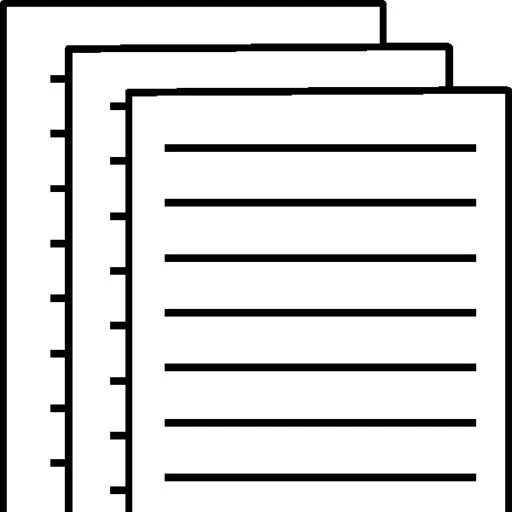## Law of demand

Law of demand

What could explain the simultaneous increases in the price of lithium and the production of lithium? Use supply and demand curves to explain your answer. (Hint: Price and equilibrium quantity have both increased. Would a shift in the demand curve or a shift in the supply curve lead to this result?)

A supply curve leads to a change in the cost of production in addition to other related factors. It affects the quantity of goods produced at a certain price. The ceteris paribus links prices to quantities supplied assuming that other factors remain constant (Cardenas, 2016). This curve shows how price affects the quantity of goods or services supplied if other factors are held constant. The curve shifts as quantity supplied changes at each price. In addition, the equilibrium shows the intersection between the supply and demand curves and it shows how a shift in the supply or demand affects the price or production of goods.

If the supply goes up, then this means that the supply curve shifts to the right and this translates to the equilibrium price decreasing as the quantity increases. If the supply decreases, then the equilibrium price goes up as the quantity decreases. On the other hand, if the demand increases, then the curve shifts to the right and if the supply and other factors remain constant, then equilibrium price and quantity simultaneously increase (Li, 2018). Therefore, the price and production of lithium simultaneously increased because the demand increased. If the demand increases over the years then the price and quantity produced are bound to increase or even double with time. However, if the demand is affected whereby people no longer want to purchase lithium, then the price will decrease as the supply decreases.

References

Cardenas, L. M., Franco, C. J., & Dyner, I. (2016). Assessing emissions–mitigation energy policy under integrated supply and demand analysis: the Colombian case. Journal of Cleaner Production112, 3759-3773.

Li, L., Chi, T., Hao, T., & Yu, T. (2018). Customer demand analysis of the electronic commerce supply chain using Big Data. Annals of Operations Research268(1-2), 113-128.

To view and download a complete answer, scroll down to the bottom to pay